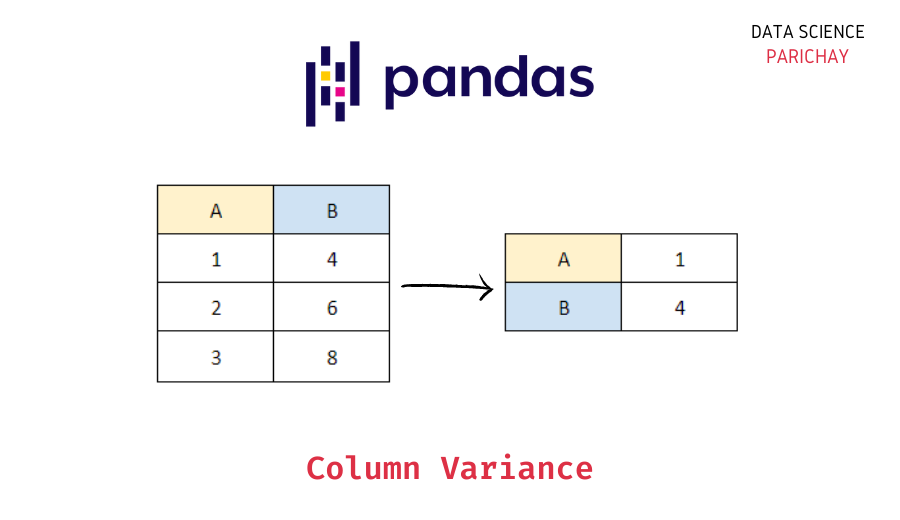# Pandas – Get Variance of One or More Columns

In this tutorial, we will look at how to get the variance of one or more columns in a pandas dataframe with the help of some examples.

You can use the pandas series `var()` function to get the variance of a single column or the pandas dataframe `var()` function to get the variance of all numerical columns in the dataframe. The following is the syntax:

```# variance of single column
df['Col'].var()
# variance of all numerical columns in dataframe
df.var()```

Let’s create a sample dataframe that we will be using throughout this tutorial to demonstrate the usage of the methods and syntax mentioned.

```import pandas as pd

# create a dataframe
df = pd.DataFrame({
'sepal_length': [5.1, 4.9, 4.7, 4.6, 5.0, 5.4, 4.6, 5.0],
'sepal_width': [3.5, 3.0, 3.2, 3.1, 3.6, 3.9, 3.4, 3.4],
'petal_length': [1.4, 1.4, 1.3, 1.5, 1.4, 1.7, 1.4, 1.5],
'petal_width': [0.2, 0.2, 0.2, 0.2, 0.2, 0.4, 0.3, 0.2],
'sepices': ['setosa']*8
})
# display the dataframe
print(df)```

Output:

```   sepal_length  sepal_width  petal_length  petal_width sepices
0           5.1          3.5           1.4          0.2  setosa
1           4.9          3.0           1.4          0.2  setosa
2           4.7          3.2           1.3          0.2  setosa
3           4.6          3.1           1.5          0.2  setosa
4           5.0          3.6           1.4          0.2  setosa
5           5.4          3.9           1.7          0.4  setosa
6           4.6          3.4           1.4          0.3  setosa
7           5.0          3.4           1.5          0.2  setosa```

The sample dataframe is taken form a section of the Iris dataset. This sample has petal and sepal dimensions of eight data points of the “Setosa” species.

You can use the pandas series `var()` function to get the variance of individual columns (which essentially are pandas series). For example, let’s get the variance of the “sepal_length” column in the above dataframe.

```# variance of sepal_length column
print(df['sepal_length'].var())```

Output:

`0.07553571428571436`

You see that we get the variance of the values in the “sepal_length” column as a scaler value.

📚 Data Science Programs By Skill Level

Introductory

Intermediate ⭐⭐⭐

🔎 Find Data Science Programs 👨‍💻 111,889 already enrolled

Disclaimer: Data Science Parichay is reader supported. When you purchase a course through a link on this site, we may earn a small commission at no additional cost to you. Earned commissions help support this website and its team of writers.

For this, first, create a dataframe with the columns that you want to calculate the variance for and then apply the pandas dataframe `var()` function. For example, let’s get the variance of the columns “sepal_length” and “sepal_width”.

```# variance of more than one columns
print(df[['sepal_length', 'sepal_width']].var())```

Output:

```sepal_length    0.075536
sepal_width     0.084107
dtype: float64```

We get the result as a pandas series. Here, we first created a subset of the dataframe “df” with only the columns “sepal_length” and “sepal_width” and then applied the `var()` function.

To get the variance of all the columns, use the same method as above but this time on the entire dataframe. Let’s use this function on the dataframe “df” created above.

```# variance of all the columns
print(df.var())```

Output:

```sepal_length    0.075536
sepal_width     0.084107
petal_length    0.014286
petal_width     0.005536
dtype: float64```

You can see that we get the variance of all the numerical columns present in the dataframe.

For more on the pandas series var() function, refer to its documentation.

Variance is a measure of spread in the data but standard deviation, the square root of variance is more generally used (as a measure of spread) since it is in the same units as the data. You can use methods similar to the ones described in this tutorial to find the standard deviation of pandas columns.

With this, we come to the end of this tutorial. The code examples and results presented in this tutorial have been implemented in a Jupyter Notebook with a python (version 3.8.3) kernel having pandas version 1.0.5

•